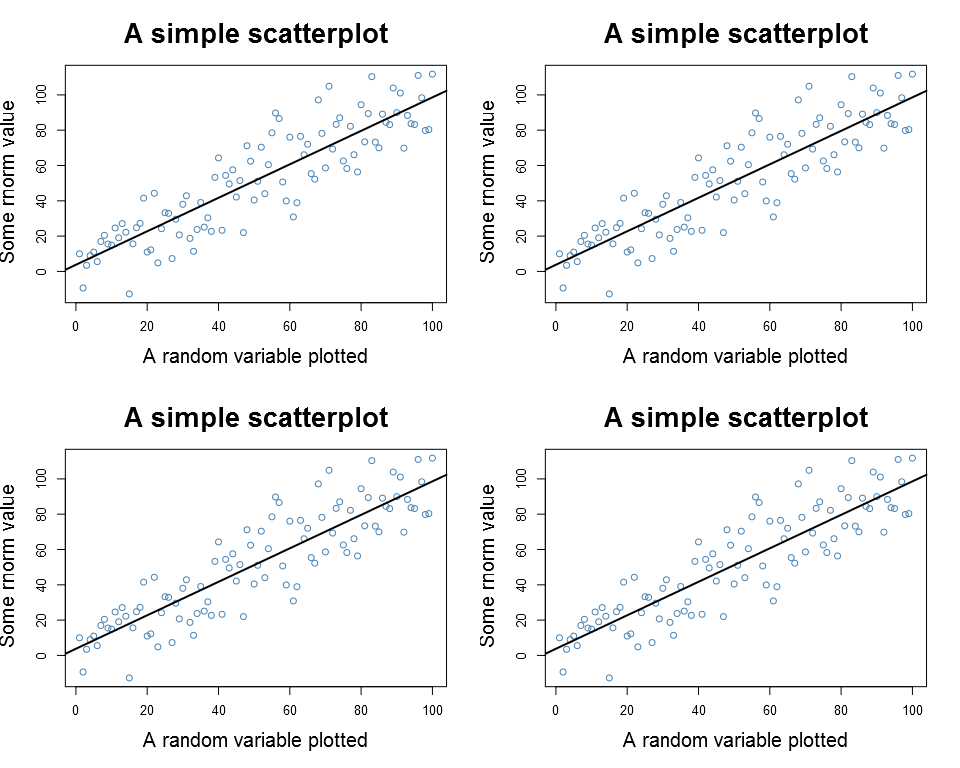Want to share your content on R-bloggers? click here if you have a blog, or here if you don't.

In a recent post I compared the Cairo packages with the base package for exporting graphs. Matt Neilson was kind enough to share in a comment that the Cairo library is now by default included in R, although you need to specify the type=”cairo” option to invoke it. In this post I examine how the ggplot and the lattice packages behave when exporting.

## Basic plot

The nice thing about the export functions in the R package is that point size scales automatically with the resolution. You don’t need to multiply the “pointsize” parameter any more, you can just go with a higher resolution and everything will look just as nice. This is very convenient as you can adjust both the text and the plotted points by just changing one export parameter. Below is an example of a plot using pointsize 12.

?View Code RSPLUS
 ```1 2 3 4 5 6 7 8 9 10 11 12 13 14 15 16 17 18 19 20 21 22 23 24 ``` ```# Create the data data <- rnorm(100, sd=15)+1:100 # Create a simple scatterplot # with long labels to enhance # size comparison my_sc_plot <- function(data){ par(cex.lab=1.5, cex.main=2) plot(data, main="A simple scatterplot", xlab="A random variable plotted", ylab="Some rnorm value", col="steelblue") x <- 1:100 abline(lm(data~x), lwd=2) } png(filename="plain_PNG_pt12.png", type="cairo", units="in", width=5, height=4, pointsize=12, res=96) my_sc_plot(data) dev.off()```

Below is the same with slightly increased point size:

?View Code RSPLUS
 ```1 2 3 4 5 6 7 8 9 ``` ```png(filename="plain_PNG_pt14.png", type="cairo", units="in", width=5, height=4, pointsize=14, res=96) my_sc_plot(data) dev.off()```

## ggplot2

This behavior is different for both the lattice and the ggplot2 packages. In both these packages the text is unchanged by the point size, although it might be good to know that the ggplot’s margins change with the point size:

?View Code RSPLUS
 ```1 2 3 4 5 6 7 8 9 10 11 12 13 14 15 16 17 18 19 20 21 22 23 24 ``` ```library(ggplot2) my_gg_plot <- function(data){ ggplot(data.frame(y=data, x=1:100), aes(y=y, x)) + geom_point(aes(x, y), colour="steelblue", size=3) + stat_smooth(method="lm", se=FALSE, color="black", lwd=1) + ggtitle("A simple scatterplot") + theme_bw() + theme(title = element_text(size=20), axis.title.x = element_text(size=18), axis.title.y = element_text(size=18), axis.text.x = element_text(size=14), axis.text.y = element_text(size=14))+ xlab("A random variable plotted") + ylab("Some rnorm value") }   png(filename="GGplot_PNG_pt12.png", type="cairo", units="in", width=5, height=4, pointsize=12, res=96) my_gg_plot(data) dev.off()```

Now notice the marigns if we compare with a plot with a the point size x 3:

?View Code RSPLUS
 ```1 2 3 4 5 6 7 8 9 ``` ```png(filename="GGplot_PNG_pt36.png", type="cairo", units="in", width=5, height=4, pointsize=12*3, res=96) my_gg_plot(data) dev.off()```

My conclusion is that there seems to be an impact on the margins but not on the text.

## Lattice

The lattice package in turn seems to be unaffected by any change to the point size:

?View Code RSPLUS
 ```1 2 3 4 5 6 7 8 9 10 11 12 13 14 15 16 17 18 19 20 21 22 23 24 ``` ```library(lattice) my_lt_plot <- function(data){ xyplot(y~x, cex=1, panel = function(x, y) { panel.xyplot(x, y) panel.abline(lm(y ~ x), lwd=2) }, lwd=2, data=data.frame(y=data, x=1:100), main=list(label="A simple scatterplot", cex=2), xlab=list(label="A random variable plotted", cex=1.8), ylab=list(label="Some rnorm value", cex=1.8), scales=list(cex=2)) }   png(filename="Lattice_PNG_pt12.png", type="cairo", units="in", width=5, height=4, pointsize=12, res=96) my_lt_plot(data) dev.off()```

And to compare with 3 times the point size:

?View Code RSPLUS
 ```1 2 3 4 5 6 7 8 9 ``` ```png(filename="Lattice_PNG_pt36.png", type="cairo", units="in", width=5, height=4, pointsize=12*3, res=96) my_lt_plot(data) dev.off()```

There seems to be no real difference.

## Panels

As a last exercise I checked what happens to the plots when using panels/side by side plots (hoping that I now covered the topic). Let’s start with the basic plot:

?View Code RSPLUS
 ```1 2 3 4 5 6 7 8 9 10 11 12 13 ``` ```png(filename="Plain_PNG_2x2.png", type="cairo", units="in", width=5*2, height=4*2, pointsize=12, res=96) par(mfrow=c(2,2)) my_sc_plot(data) my_sc_plot(data) my_sc_plot(data) my_sc_plot(data) dev.off()```Plain plot, there is a minor difference and the text seems a little smaller in the 2×2 plot

Both the ggplot and the lattice performed better:

?View Code RSPLUS
 ```1 2 3 4 5 6 7 8 9 10 11 12 13 14 ``` ```png(filename="GGplot_PNG_2x2.png", type="cairo", units="in", width=5*2, height=4*2, pointsize=12, res=96) p1 <- my_gg_plot(data) pushViewport(viewport(layout = grid.layout(2, 2))) print(p1, vp = viewport(layout.pos.row = 1, layout.pos.col = 1)) print(p1, vp = viewport(layout.pos.row = 1, layout.pos.col = 2)) print(p1, vp = viewport(layout.pos.row = 2, layout.pos.col = 1)) print(p1, vp = viewport(layout.pos.row = 2, layout.pos.col = 2)) dev.off()```
?View Code RSPLUS
 ```1 2 3 4 5 6 7 8 9 10 11 12 13 ``` ```png(filename="Lattice_PNG_2x2.png", type="cairo", units="in", width=5*2, height=4*2, pointsize=12, res=96) p1 <- my_lt_plot(data) print(p1, position=c(0, .5, .5, 1), more=TRUE) print(p1, position=c(.5, .5, 1, 1), more=TRUE) print(p1, position=c(0, 0, .5, .5), more=TRUE) print(p1, position=c(.5, 0, 1, .5)) dev.off()```